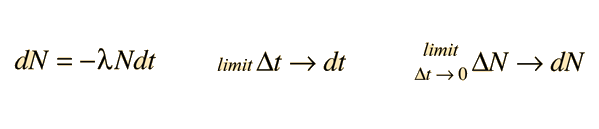# Limits

Most of the applications of calculus deal with continuous functions, but the visualization of processes often starts with finite changes in the variables of interest. One power of calculus is that it can deal with changes which are infinitesmally small. For example, an average velocity is obtained by taking a distance traveled and dividing by the finite time interval it took to travel that distance. But suppose you wanted the instantaneous velocity at a given instant of time. You can approach that with a "limit", i.e., you can take the smaller distances traveled in shorter intervals and divide by those shorter times. If this process is allowed to continue until the time interval approaches zero, then this is called "taking the limit as the time interval approaches zero". This is the way a derivative of distance with respect to time is defined and the way that an instantaneous velocity is calculated using calculus methods. Limits are also used in the formation of integrals, or anti-derivatives.
Index

 HyperPhysics*****HyperMath*****Calculus R Nave
Go Back

# Differential Form

As a precursor to carrying out calculus operations such as derivatives and integrals, a mathematical expression will have to be placed in the differential form for the application of the methods of continuous variables. For example, a finite difference expression occurs in the development of the relationship for radioactive decay:It is standard practice to use the Greek letter Δ to represent a finite difference. Often the finite difference relationship is only approximately true and is exactly true only in the limit where the differences become infinitesmally small. In this limit, the time interval Δt becomes vanishingly small and as a result, the number of decays N also becomes vanishingly small. The Δ is replaced by the differential symbol d and the resulting formis said to be the differential form of the expression.